Name:    Chapter 8 Post Test

Multiple Choice
Identify the choice that best completes the statement or answers the question.

1.

Determine the order of the matrix.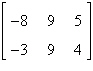a. 2 ´ 3 b. 2 ´ 2 c. 3 ´ 3 d. 3 ´ 2 e. 3 ´ 1

2.

Write the system of linear equations represented by the augmented matrix. (Use variables x, y, z, and w.)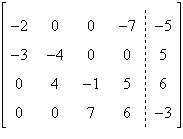a.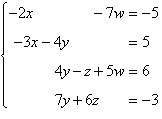b.c.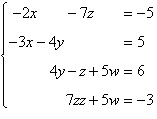d.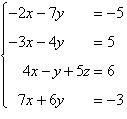e.3.

Fill in the blank using elementary row operations to form a row-equivalent matrix.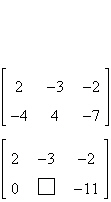a.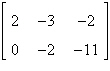b.c.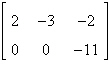d.e.4.

Use matrices to solve the system of equations (if possible). Use Gaussian elimination with back-substitution or Gauss-Jordan elimination.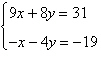a. x = 5, y = 1 b. x = 5, y = –1 c. no solution d. x = 1, y = –5 e. x = –1, y = 5

5.

Use matrices to solve the system of equations (if possible). Use Gaussian elimination with back-substitution or Gauss-Jordan elimination.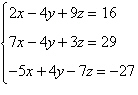a. x = 5, y = 3, z = 2 b. x = 5, y = –3, z = 2 c. x = –5, y = –3, z = 2 d. no solution e. x = 3, y = –5, z = –2

6.

Evaluate the expression.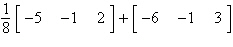a.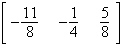b. not possible c.d.e.7.

Solve for X in the equation given.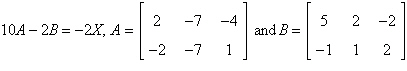a.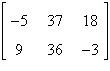b.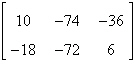c.d. not possible e.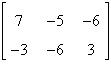8.

If possible, find AB.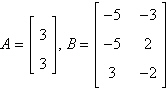a.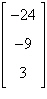b. not possible c.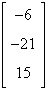d.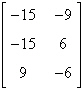e.9.

Use the matrix capabilities of a graphing utility to find AB, if possible.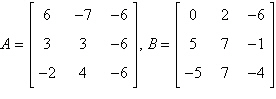a. not possible b.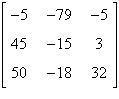c.d.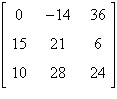e.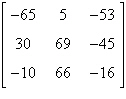10.

Of the products AB, BA, A2, and B2, which ones are possible for the given matrices?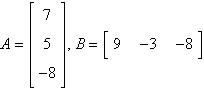a. A2 only b. BA only c. B2 only d. AB and BA only e. AB only

11.

Evaluate the expression.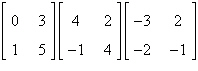a.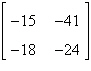b.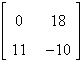c.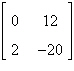d. not possible e.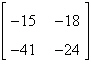12.

Find the inverse of the matrix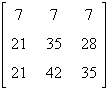.
 a.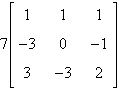b.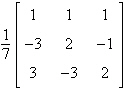c.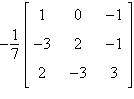d.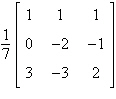e.13.

Solve the system of linear equations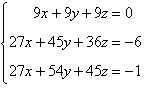using the inverse matrix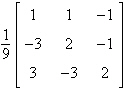.
 a.b.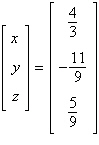c.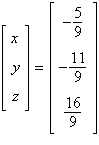d.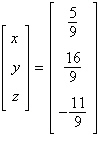e.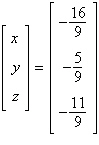14.

Solve the system of linear equationsusing the inverse matrix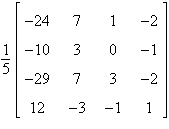.
 a.d.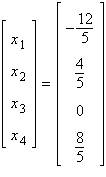b.e.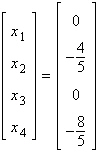c.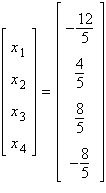15.

Solve the system of linear equations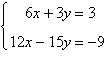using an inverse matrix.
 a.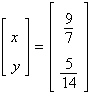b.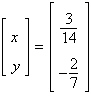c.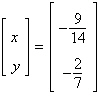d.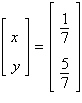e.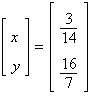16.

Use the matrix capabilities of a graphing utility to solve the following system of linear equations: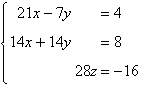a.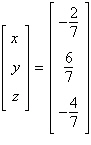b.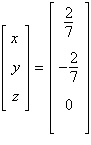c.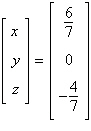d.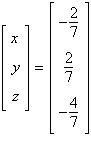e.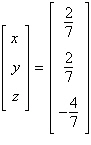17.

Use the matrix capabilities of a graphing utility to find the determinant of the matrix.
 a.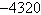b. –1080 c. –432 d.e.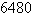18.

Use the matrix capabilities of a graphing utility to find the determinant of the matrix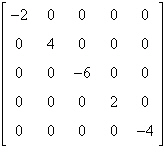.
 a. –384 b. –32 c. –64 d. –48 e. 128

19.

Given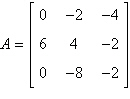, find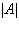.
 a. 88 b. –120 c. 168 d. 40 e. –80

20.

You inherited a triangular piece of property after your Uncle Izzy passed away. You want to know the size of land, so you "step it off" to estimate the square footage. From the southernmost vertex A, you travel north 220 feet then west 280 feet (for vertex C), and from the southernmost vertex A, you travel 520 feet north then 110 feet west (for vertex B). Use a graphing utility to approximate the number of square feet of land that you have inherited.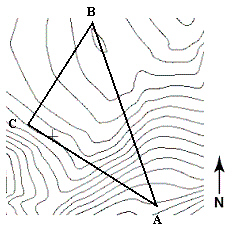a. 60,700 square feet b.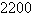square feet c. 59,400 square feet d. 84,900 square feet e.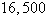square feet